# How to draw epicycloid and hypocycloid movements

What is an epicycloid?

 ...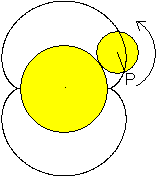... A (generating) circle with the radius R is given. If you roll a second circle with the radius r on it and follow the path of a point P on the circular line, the epicycloid is created.In this case, R = 2r or 2pi * R = 2 * (2pi * r).Then there is a simply closed curve, the nephroids.

The curve is more intricate when the ratio R: r is no longer an integer.
 ...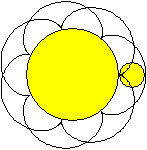If the relationship is rational, the curve closes after a number of revolutions.Here is R: r = 7: 2. The curve closes after two laps.

 ...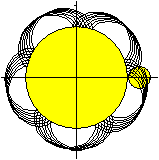If the ratio is irrational, such as R: r = 7: sqrt (2), the curve does not close.

These epicycloids have one property in common: They lie in a circular ring, which is formed by the generating circle with the radius R and an enveloping circle with the radius R + 2r.

Parametric representation of the epicycloids Top
The epicycloids on this page are created using a parametric display with the program Winplot drawn. The formulas are

x = (R + r) cos (t) -r cos [(1 + R / r) t]
y = (R + r) sin (t) -r sin [(1 + R / r) t]
We have D = | R.

Derivation of the formulas
 ...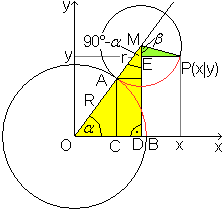Because of the rolling condition, the arcs over AB and AP are the same.The following applies: b (AB) = (pi * alpha * R) / 180 ° and b (AP) = [pi * (90 ° -alpha + beta) * r] / 180 °.This implies alpha * R = (90 ° -alpha + beta) * r or beta = (1 + R / r) alpha-90 °.In the yellow triangle ODM appliessin (alpha) = MD / OM or MD = (R + r) * sin (alpha) andcos (alpha) = OD / OM or OD = (R + r) * cos (alpha).In the green triangle EPM appliessin (beta) = EP / MP or EP = r * sin (beta)cos (beta) = ME / MP or ME = r * cos (beta)
Next is
x = OD + EP = (R + r) * cos (alpha) + r * sin (beta) = (R + r) * cos (alpha) + r * sin [(1 + R / r) alpha-90 ° ]
= (R + r) * cos (alpha) -r * cos [(1 + R / r) alpha]
y = MD-ME = (R + r) * sin (alpha) -r * cos (beta) = (R + r) * sin (alpha) -r * cos [(1 + R / r) alpha-90 ° ]
= (R + r) * sin (alpha) -r * sin [(1 + R / r) alpha].

It is common practice to specify the angles in radians. So we set alpha = t and get
x = (R + r) cos (t) -r cos [(1 + R / r) t]
y = (R + r) sin (t) -r sin [(1 + R / r) t].

Simply closed curves Top
Of interest is the simply closed curve that results when the ratio of the radii of the two circles involved is an integer. In this case one likes to insert R / r = m into the parameter equations.

 x = (R + r) cos (t) -r cos [(1 + R / r) t]y = (R + r) sin (t) -r sin [(1 + R / r) t]. x = (m + 1) r cos (t) -r cos [(m + 1) t]y = (m + 1) r sin (t) -r sin [(m + 1) t]

ExampleD = {t | 0 <= t <= 2pi}

Description of the curves
 ..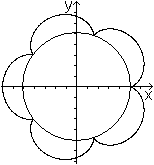.... > They consist of m congruent arcs that curve over the generating circle.> For the angles 2 (k-1) pi / m (1 <= k> = m) there are peaks that lie on the circle with the radius R.> For the angles 2 [(k-1) / 2] pi / m (1 <= k> = m) there are vertices that lie on a circle with the radius R + 2r.> The adjacent epicycloid is represented byx = 6cos (t) -cos (6t)y = 6sin (t) -sin (6t)

Area and perimeter Top
For the simply closed epicycloid the question arises as to the area and the circumference.

Cardioids
On my Heart Curve page I show how to determine it for the cardioids (m = 1).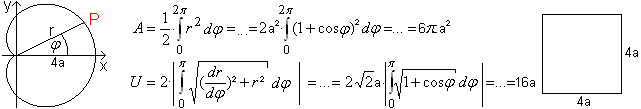This procedure cannot be generalized, since there is apparently no corresponding polar equation for the epicycloids for the general case. (At Mathworld / Epicycloid one finds an equivalent equation.)

General formulas
In the literature one finds the formulas A = (m + 1) (m + 2) pi * r² and U = 8 (m + 1) r.

Application to simple epicycloids
 Cardioidsm = 1A = 6pi * r²U = 16r Nephroidsm = 2A = 12pi * r²U = 24r Triple-beam epicycloidm = 3A = 20pi * r²U = 32r Four-pointed epicycloidsm = 4A = 30pi * r²U = 40r Five-pointed epicycloidsm = 5A = 42pi * r²U = 48r

Another reading of the formula for area
 ...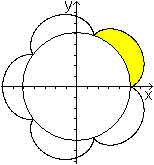... In book (3) you can find the formula for the area between the generating circle and an arc.A '= pi * r² (3R + 2r) / R.The total area is thenA = mA '+ pi * R² = m [pi * r² (3R + 2r) / R] = ... = pi * r² (m² + 3m + 2) = (m + 1) (m + 2) pi * r² as stated above.

To derive the formula A = (m + 1) (m + 2) pi * r².
For the calculation of the area the Leibniz sector formula ready.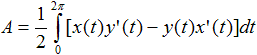x (t) = (m + 1) r cos (t) -r cos [(m + 1) t]y (t) = (m + 1) r sin (t) -r sin [(m + 1) t] x '(t) = - (m + 1) r sin (t) + r (m + 1) sin [(m + 1) t]y '(t) = (m + 1) r cos (t) -r (m + 1) cos [(m + 1) t]

We have x (t) y '(t) -y (t) x' (t)
= (m + 1) ²r² [sin² (t) + cos² (t)] + (m + 1) r² {sin² [(m + 1) t] + cos² [(m + 1) t]}
- [(m + 1) ²r² + (m + 1) r²] {sin (t) sin [(m + 1) t] + cos (t) cos [(m + 1) t]}
= (m + 1) ²r² + (m + 1) r² - [(m + 1) ²r² + (m + 1) r²] cos (mt)
= [(m + 1) ²r² + (m + 1) r²] [1-cos (mt)]
= (m + 1) (m + 2) r² [1-cos (mt)]
Then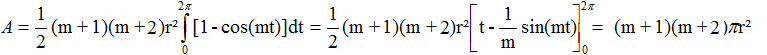, wzbw.

To derive the formula U = 8 (m + 1) r
 ...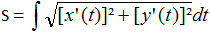... The formula opposite is available for the length of an arc.
We have [x '(t)] ² + [y' (t)] ²
= {- (m + 1) r sin (t) + r (m + 1) sin [(m + 1) t]} ² + {(m + 1) r cos (t) -r (m + 1) cos [(m + 1) t]} 2
= (m + 1) ²r² + (m + 1) ²r²-2 (m + 1) ²r² {sin (t) sin [(m + 1) t] + cos (t) cos [(m + 1) t] }
= 2 (m + 1) ²r²-2 (m + 1) ²r²cos (mt)
= (m + 1) 2 r 2 [2-2cos (mt)].
Then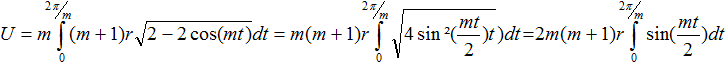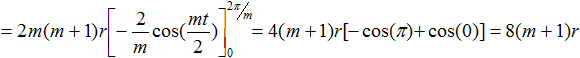, wzbw.

Multiple revolving curves Top
The curve is more intricate when the ratio R: r is no longer an integer.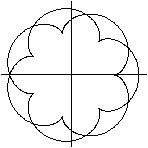If the ratio is rational, such as R: r = 7: 2, the curve closes after a number of revolutions.The parametric equations are herex = 9cos (t) -2cos [(9/2) t]y = 9sin (t) -2sin [(9/2) t]D = {t | 0
If the ratio R: r = p / q, where p and q are prime numbers, then the curve closes after q revolutions.

Examples

Not closed curves Top

 ...If the ratio is irrational, such as R: r = 7: sqrt (2), the curve does not close.x = 8.414cos (t) -1.414cos [8.414 / 1.414) t]y = 8.414sin (t) -1.414sin [(8.414 / 1.414) t]0

Arcs bulge over the generating circle. As the parameter increases, they move counter-clockwise. This is also shown in the following series of images.

Epitrochoid Top
Common Epicycloids (or Common E.)

 ...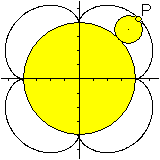So far, a point P was followed when rolling on the circular line of the rolling circle.The common epicycloid arises.

Points that do not lie on the circular line also describe a closed curve, the Epitrochoid.

A distinction is made between whether the point P lies inside or outside the rolling circle.

In both cases the curves are captured by the slightly modified parametric equations from above.

x = (R + r) cos (t) -a cos [(1 + R / r) t]
y = (R + r) sin (t) -a sin [(1 + R / r) t]
The variable a indicates the distance of the point P from the center of the rolling circle. So far, a = r.

Elongated Epicycloids (or Shortened E.)
The point P lies within of the rolling circle at a distance a from its center.
 ...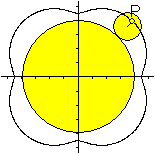...a = 0.5r Compared to the usual epicycloids, the tips become indentations.The vertices and indentations are smoothed and the epicycloid degenerates into a circle when the variable a approaches zero.

Tangled Epicycloids (or Elongated E.)
The point P lies outside of the circle at a distance a from its center.
 ...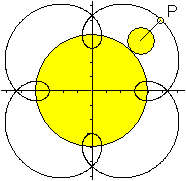...a = 2r As the name suggests, the intertwined epicycloid has loops.These become larger, the larger the variable a is.They finally overlap so far that they form inside m-corners from arches.This is shown in the following series of images.

Epicycloids follow for m = 3 and for various parameters a.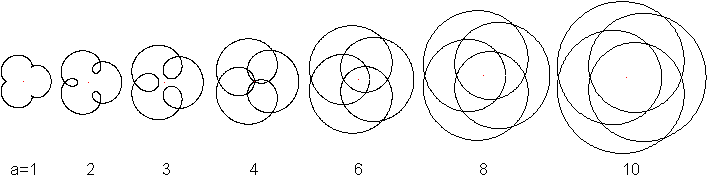Epicycloids follow for m = 4 and for various parameters a.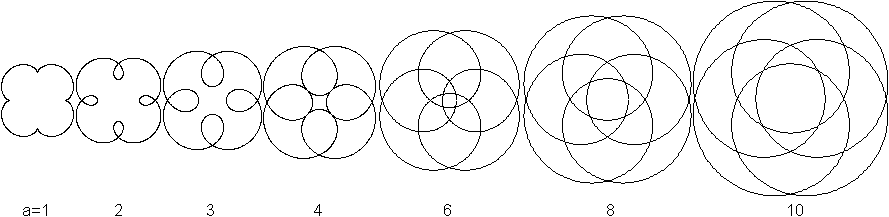Hypocycloid Top

 ...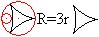... ...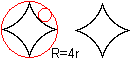... A hypocycloid arises when a rolling circle is not around, rather in moving a generating circle. In doing so, one follows the path of a circle point of the rolling circle.

The hypocycloid is also described by similar parameter equations as for epicycloids.
x = (R-r) cos [(r / R) t] + a cos [(1-r / R) t]
y = (R-r) sin [(r / R) t] -a sin [(1-r / R) t]

The equations are derived and applied on my Spirograph website.

Some examples

Alienation of the parametric equations Top

Most of the time, the figures were rotated so that the axis of symmetry was vertical.

The pictures from above now with color

Spirograph Top

 ...... Epi- and hypocycloids form the basis of a widely used toy, the spirograph.

Epicycloids on the Internet Top

German

Norbert Treitz
Animations for epi- and hypotrochoid

Stefan Huebbers
Consideration of epicycloids

Department of Physics (University of Innsbruck)
Lissajous apparatus

Wikipedia
Epicycloids, Cardioids, Nephroids, Cycloids, Leibniz Sector Formula, Spirograph (Toys)

English

Eric W. Weisstein (MathWorld)
Epicycloid, Cardioid, Nephroid, Ranunculoid, Tusi Couple

Famous Curves Index
Epicycloid, Cardioid, Nephroid, Hypocycloid

Gerd Breitenbach
Curves of planetary motion in geocentric perspective: Epitrochoids

Richard Parris (freeware program WINPLOT)
The official website is closed. Download the German program e.g. from heise

Saltire software
Area Enclosed by a General Hypocycloid

Wikipedia
Epicycloid, Cardioid, Nephroid, Epitrochoid, Hypocycloid, Spirograph
Xahlee
Epicycloid and Hypocycloid, Epitrochoid, Hypotrochoid

Marius Mikucionis
Epitrochoid generator

David Little
SpiroGraph

French

Robert FERRÉOL, (Mathcurve)
ÉPICYCLOÏDE, CYCLOÏDE SPHÉRIQUE, HYPOCYCLOÏDE

credentials Top
(1) Wilhelm Leupold (among others): Analysis for engineering and technical schools, Frankfurt / M Zurich 1966
(2) Heinz Nickel (among others): Algebra and Geometry for Engineering and Technical Schools, Frankfurt / M Zurich 1966
(3) W. Gellert (Ed.): Kleine Enzyklopädie Mathematik, Leipzig 1986

Feedback: Email address on my main page

URL of my homepage:
http://www.mathematische-basteleien.de/